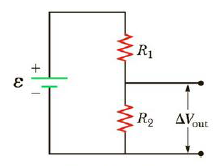Chapter 18, Problem 26P

Chapter
Section
Textbook Problem

Figure P18.26 shows a voltage divider, a circuit used to obtain a desired voltage ΔVout from a source voltage ε. Determine the required value of R2 if ε = 5.00 V, ΔVout = 1.50 V and R1 = 1.00 × 103 Ω (Hint: Use Kirchhoff's loop rule, substituting ΔVout = IR2, to find the current. Then solve Ohm’s law for R2.Figure P18.26

To determine
The current I in the circuit and resistance R2 by using ohm’s law.

Explanation

Given Info: Source voltage ε is 5.00V , desired voltage ΔVout is 1.50V Resistance R1 is 1.00×103Ω , desired voltage in terms of I is Vout is IR2 .

Explanation:

Apply Kirchhoff’s loop rule

εIR1Vout=0

On rearrange

εIR1Vout=0I=εVoutR1

Formula to calculate the current I in the circuit is

I=εVoutR1

• I is the current in the circuit
• ε is the source voltage
• R1 is the Resistance
• Vout is the desired voltage obtain from source voltage.

Substitute 5.00V for ε and 1.00×103Ω for R1 and 1.50V for ΔVout to find I

I=5

Still sussing out bartleby?

Check out a sample textbook solution.

See a sample solution

The Solution to Your Study Problems

Bartleby provides explanations to thousands of textbook problems written by our experts, many with advanced degrees!

Get Started